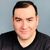# App Development

Discussion board where members can learn more about Qlik Sense App Development and Usage.

cancel
Showing results for
Did you mean:Contributor II

## Fonction random

Bonjour la communauté Qlik,

J'ai une question concernant la fonction random.

J'aimerais générer des lignes aléatoire sur les ventes de chaussures mais lorsque j'utilise la fonction random, elle me renvoie une distribution linéaire. Lorsque je charge mes données, j'obtiendrai toujours le même ordre de classement des marques de chaussures.

Ci dessous,  mon code :

LET vJOURS = '25' ;
LET vMOIS = '7' ;
LET vANNEES = '5' ;
LET vMODELE = '40' ;
LET vBOUTIQUE = '44' ;
LET vAGE = '45' ;
LET vPAYS = '5' ;
LET vVILLE = '2' ;
LET vCOULEUR = '3' ;
LET vTAILLE = '32' ;
LET vPRIX = '81' ;
LET vA = 0;

DO WHILE vA < 5

Ventes:
ROUND(RAND()*'\$(vJOURS)'+'\$(vA)')+1 as JOURS,
ROUND(RAND()*'\$(vMOIS)')+'\$(vA)'+1 as MOIS,
FABS(ROUND((sqrt(-2*log(rand())) * cos(2* pi() * rand())* 2)+3)) as ID_ANNEES,
FABS(ROUND((sqrt(-2*log(rand())) * sin(2* pi() * rand())*+8)+43)) as ID_MODELES,
FABS(ROUND((sqrt(-2*log(rand())) * tan(2* pi() * rand())*10)+45)) as ID_BOUTIQUE,
FABS(ROUND(RAND()*'\$(vA)')-2) as ID_SEXE,
ROUND(RAND()*'\$(vTAILLE)'-'\$(vA)')+'\$(vA)'+1 as ID_TAILLE,
'1' as CNT
Autogenerate ROUND(RAND()*800000);

LET vA = vA+1;
LOOP

Avez-vous une solution pour palier à ce problème afin que je puisse obtenir une réelle distribution aléatoire ?

Merci pour votre aide,

Lucie

2 RepliesCreator

Bonjour. Rand () est une distribution normale et tend donc à niveler les résultats.

Vous pouvez essayer d'utiliser ce qui suit pour pondérer les nombres faibles:
Rand () * Rand () * N
en remplaçant N par différentes constantes de votre choix.

Pour quelques résultats possibles, vous pouvez également utiliser quelque chose comme ceci:
if (Rand () <0.6, 'F', 'M') // pour 60% de chances d'obtenir 'F' et 40% 'M'

Cordialement, Fernando
(désolé mon français de google translate)Specialist II

hi!, here you have a code from qlikview,, check if the rand() parts works for you.

Characters:
Load Chr(RecNo()+Ord('A')-1) as Alpha, RecNo() as Num autogenerate 26;

ASCII:
if(RecNo()>=65 and RecNo()<=90,RecNo()-64) as Num,
Chr(RecNo()) as AsciiAlpha,
RecNo() as AsciiNum
autogenerate 255
Where (RecNo()>=32 and RecNo()<=126) or RecNo()>=160 ;

Transactions:
TransLineID,
TransID,
mod(TransID,26)+1 as Num,
Pick(Ceil(3*Rand1),'A','B','C') as Dim1,
Pick(Ceil(6*Rand1),'a','b','c','d','e','f') as Dim2,
Pick(Ceil(3*Rand()),'X','Y','Z') as Dim3,
Round(1000*Rand()*Rand()*Rand1) as Expression1,
Round( 10*Rand()*Rand()*Rand1) as Expression2,
Round(Rand()*Rand1,0.00001) as Expression3;